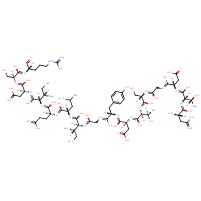Structure InformationCompound Identification

SMILES

CC[C@H](C)[C@H](NC(=O)CNC(=O)[C@H](CC1=CC=C(O)C=C1)NC(=O)[C@H](CC(O)=O)NC(=O)[C@@H](NC(=O)[C@H](CO)NC(=O)CNC(=O)[C@H](CC(O)=O)NC(=O)[C@@H](NC(=O)[C@@H](N)CC(N)=O)[C@@H](C)O)[C@@H](C)O)C(=O)N[C@@H](CC(C)C)C(=O)N[C@@H](CCC(N)=O)C(=O)N[C@@H]([C@@H](C)CC)C(=O)N[C@@H](CC(N)=O)C(=O)N[C@@H](CO)C(=O)N[C@@H](CCCN=C(N)N)C=O

InChIKey

InChIKey=TYIZBCRZPLSZKH-VGYLNWHASA-N

Formula

C72H116N22O28

Mass

1737.846

### Export to:

Taxonomic Classification

Taxonomy Tree

Kingdom

Organic compounds

Superclass

Organic Polymers

Class

Polypeptides

Subclass

Not available

Intermediate Tree Nodes

Not available

Direct Parent

Polypeptides

Alternative Parents

Molecular Framework

Aromatic homomonocyclic compounds

Substituents

Polypeptide - Alpha peptide - Tyrosine or derivatives - Phenylalanine or derivatives - Glutamine or derivatives - Asparagine or derivatives - Aspartic acid or derivatives - Isoleucine or derivatives - Leucine or derivatives - N-acyl-alpha amino acid or derivatives - Alpha-amino acid amide - Serine or derivatives - N-substituted-alpha-amino acid - Amphetamine or derivatives - Alpha-amino acid or derivatives - Phenol - 1-hydroxy-2-unsubstituted benzenoid - N-acyl-amine - Fatty acyl - Fatty amide - Dicarboxylic acid or derivatives - Monocyclic benzene moiety - Benzenoid - Amino acid or derivatives - Primary carboxylic acid amide - Secondary alcohol - Secondary carboxylic acid amide - Carboxamide group - Amino acid - Guanidine - Organic 1,3-dipolar compound - Propargyl-type 1,3-dipolar organic compound - Carboximidamide - Carboxylic acid derivative - Carboxylic acid - Organic oxide - Organic oxygen compound - Organic nitrogen compound - Carbonyl group - Aldehyde - Alcohol - Hydrocarbon derivative - Amine - Primary amine - Primary alcohol - Organooxygen compound - Primary aliphatic amine - Organonitrogen compound - Aromatic homomonocyclic compound

Description

This compound belongs to the class of organic compounds known as polypeptides. These are peptides containing ten or more amino acid residues.

External Descriptors

Not available